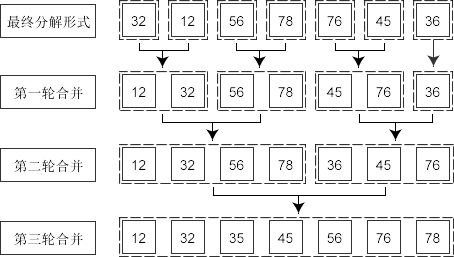# C语言归并排序（合并排序）算法及代码

(1)将所要进行的排序序列分为左右两个部分，如果要进行排序的序列的起始元素下标为first，最后一个元素的下标为last，那么左右两部分之间的临界点下标mid=(first+last)/2，这两部分分别是A[first … mid]和A[mid+1 … last]。

(2)将上面所分得的两部分序列继续按照步骤(1)继续进行划分，直到划分的区间长度为1。

(3)将划分结束后的序列进行归并排序，排序方法为对所分的n个子序列进行两两合并，得到n/2或n/2+l个含有两个元素的子序列，再对得到的子序列进行合并，直至得到一个长度为n的有序序列为止。下面通过一段代码来看如何实现归并排序。

1. #include <stdio.h>
2. #include <stdlib.h>
3. #define N 7
4.
5. void merge(int arr[], int low, int mid, int high){
6. int i, k;
7. int *tmp = (int *)malloc((high-low+1)*sizeof(int));
8. //申请空间，使其大小为两个
9. int left_low = low;
10. int left_high = mid;
11. int right_low = mid + 1;
12. int right_high = high;
13.
14. for(k=0; left_low<=left_high && right_low<=right_high; k++){ // 比较两个指针所指向的元素
15. if(arr[left_low]<=arr[right_low]){
16. tmp[k] = arr[left_low++];
17. }else{
18. tmp[k] = arr[right_low++];
19. }
20. }
21.
22. if(left_low <= left_high){ //若第一个序列有剩余，直接复制出来粘到合并序列尾
23. //memcpy(tmp+k, arr+left_low, (left_high-left_low+l)*sizeof(int));
24. for(i=left_low;i<=left_high;i++)
25. tmp[k++] = arr[i];
26. }
27.
28. if(right_low <= right_high){
29. //若第二个序列有剩余，直接复制出来粘到合并序列尾
30. //memcpy(tmp+k, arr+right_low, (right_high-right_low+1)*sizeof(int));
31. for(i=right_low; i<=right_high; i++)
32. tmp[k++] = arr[i];
33. }
34.
35. for(i=0; i<high-low+1; i++)
36. arr[low+i] = tmp[i];
37. free(tmp);
38. return;
39. }
40.
41. void merge_sort(int arr[], unsigned int first, unsigned int last){
42. int mid = 0;
43. if(first<last){
44. mid = (first+last)/2; /* 注意防止溢出 */
45. /*mid = first/2 + last/2;*/
46. //mid = (first & last) + ((first ^ last) >> 1);
47. merge_sort(arr, first, mid);
48. merge_sort(arr, mid+1,last);
49. merge(arr,first,mid,last);
50. }
51. return;
52. }
53.
54. int main(){
55. int i;
56. int a[N]={32,12,56,78,76,45,36};
57.
58. printf ("排序前 \n");
59. for(i=0;i<N;i++)
60. printf("%d\t",a[i]);
61.
62. merge_sort(a,0,N-1); // 排序
63.
64. printf ("\n 排序后 \n");
65. for(i=0;i<N;i++)
66. printf("%d\t",a[i]); printf("\n");
67.
68. system("pause");
69. return 0;
70. }

```排序前
32    12    56    78    76    45    36

12    32    36    45    56    76    78```(0)
0%

(0)
0%